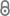# Complexity and approximation of a geometric local robot assignment problem

O. Bonorden, B. Degener, P. Pietrzyk, B. Kempkes, Complexity and Approximation of a Geometric Local Robot Assignment Problem, 2009.pub-hni-3956.pdf 258.11 KB
Report | English
Author
Bonorden, Olaf; Degener, Bastian; Pietrzyk, Peter; Kempkes, Barbara
Publishing Year
LibreCat-ID

### Cite this

Bonorden O, Degener B, Pietrzyk P, Kempkes B. Complexity and Approximation of a Geometric Local Robot Assignment Problem.; 2009.
Bonorden, O., Degener, B., Pietrzyk, P., & Kempkes, B. (2009). Complexity and approximation of a geometric local robot assignment problem.
@book{Bonorden_Degener_Pietrzyk_Kempkes_2009, title={Complexity and approximation of a geometric local robot assignment problem}, author={Bonorden, Olaf and Degener, Bastian and Pietrzyk, Peter and Kempkes, Barbara}, year={2009} }
Bonorden, Olaf, Bastian Degener, Peter Pietrzyk, and Barbara Kempkes. Complexity and Approximation of a Geometric Local Robot Assignment Problem, 2009.
O. Bonorden, B. Degener, P. Pietrzyk, and B. Kempkes, Complexity and approximation of a geometric local robot assignment problem. 2009.
Bonorden, Olaf, et al. Complexity and Approximation of a Geometric Local Robot Assignment Problem. 2009.
Main File(s)
File Name
pub-hni-3956.pdf 258.11 KB
Access LevelClosed Access